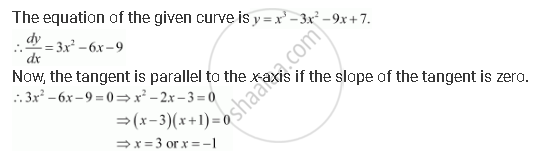Share

# Find Points at Which the Tangent to the Curve Y = X3 − 3x2 − 9x + 7 is Parallel to the X-axis. - CBSE (Science) Class 12 - Mathematics

#### Question

Find points at which the tangent to the curve y = x3 − 3x2 − 9x + 7 is parallel to the x-axis.

#### SolutionWhen x = 3, y = (3)3 − 3 (3)2 − 9 (3) + 7 = 27 − 27 − 27 + 7 = −20.

When x = −1, y = (−1)3 − 3 (−1)2 − 9 (−1) + 7 = −1 − 3 + 9 + 7 = 12.

Hence, the points at which the tangent is parallel to the x-axis are (3, −20) and

(−1, 12).

Is there an error in this question or solution?

#### APPEARS IN

NCERT Solution for Mathematics Textbook for Class 12 (2018 to Current)
Chapter 6: Application of Derivatives
Q: 7 | Page no. 211

#### Video TutorialsVIEW ALL 

Solution Find Points at Which the Tangent to the Curve Y = X3 − 3x2 − 9x + 7 is Parallel to the X-axis. Concept: Tangents and Normals.
S